# Algorithms Three types of Computational Thinking Name Three

• Slides: 19Algorithms Three types of Computational Thinking Name Three Standard Searching algorithms Explanation Three Standard Sorting algorithms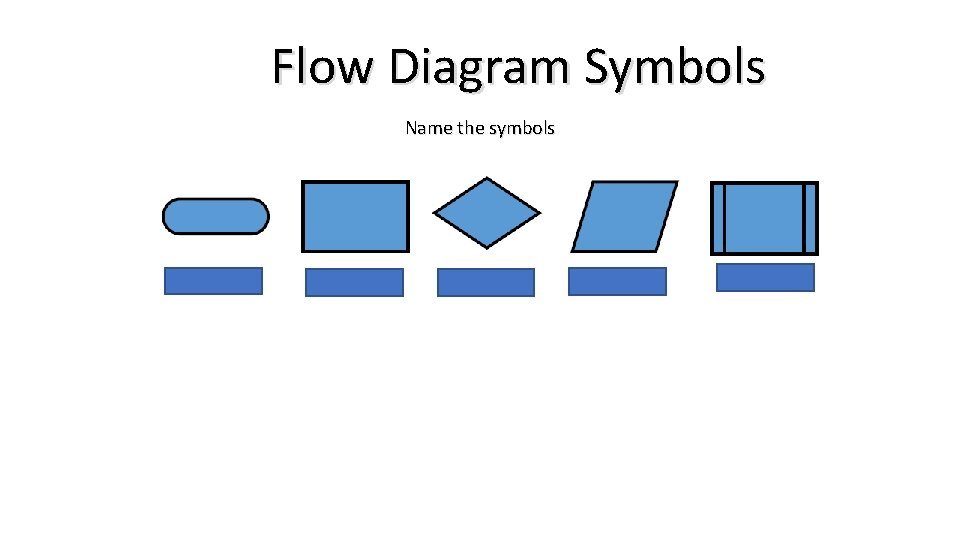Flow Diagram Symbols Name the symbolsWhat Type of search am I talking about • Used to search a list or array for a certain item and returns the index. • Can be used on unsorted lists. • Checks each item in turn one-by-one until the item is found. • If the item isn’t found a value of – 1 is returned. • Not efficient for large lists although you might get lucky and the item could be in the first few you check. What Type of search am I talking about • The array must be sorted. • A mid point is found a logical test is made to decide whether the item is less than, greater than or equal to the mid point. • If less than or greater than, half the array is discounted. This repeats until the mid point = the item found. • Uses three pointers—low, mid and high. • Much faster for large lists than linear search but array must be sorted first.What Type of SORT am I talking about involves looping through every item in an array. • Each item is compared to the one to its right. If they are in the wrong order, they swap. • A variable needs to be used to store whethere were any swaps in a pass. When there are no swaps the algorithm ends. • Each pass the largest value will MOVE to the right. This means it is not necessary to check this number again. • THIS sort is a very slow sort and there is no reason to use it other than for academic purposes. The only advantage is that some may find it slightly easier to implement than some other sorts.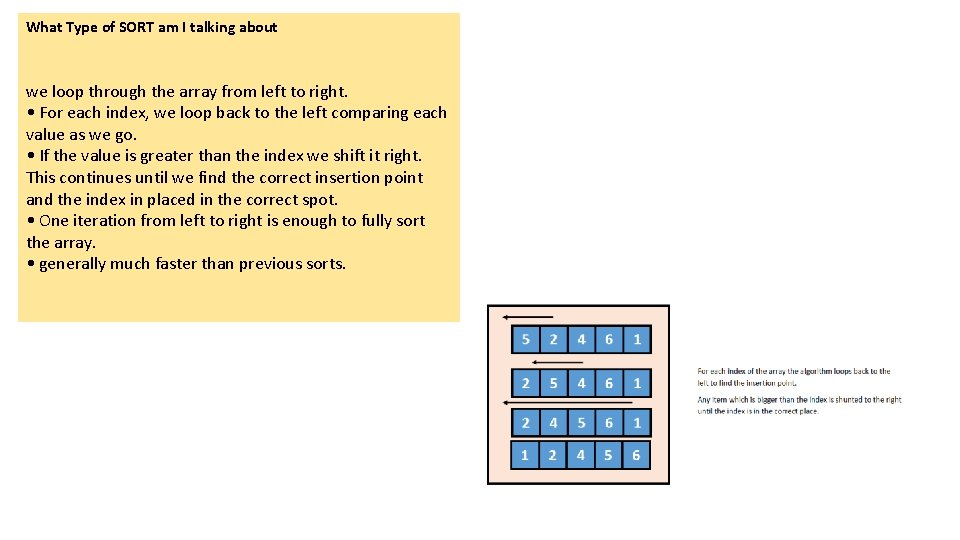What Type of SORT am I talking about we loop through the array from left to right. • For each index, we loop back to the left comparing each value as we go. • If the value is greater than the index we shift it right. This continues until we find the correct insertion point and the index in placed in the correct spot. • One iteration from left to right is enough to fully sort the array. • generally much faster than previous sorts.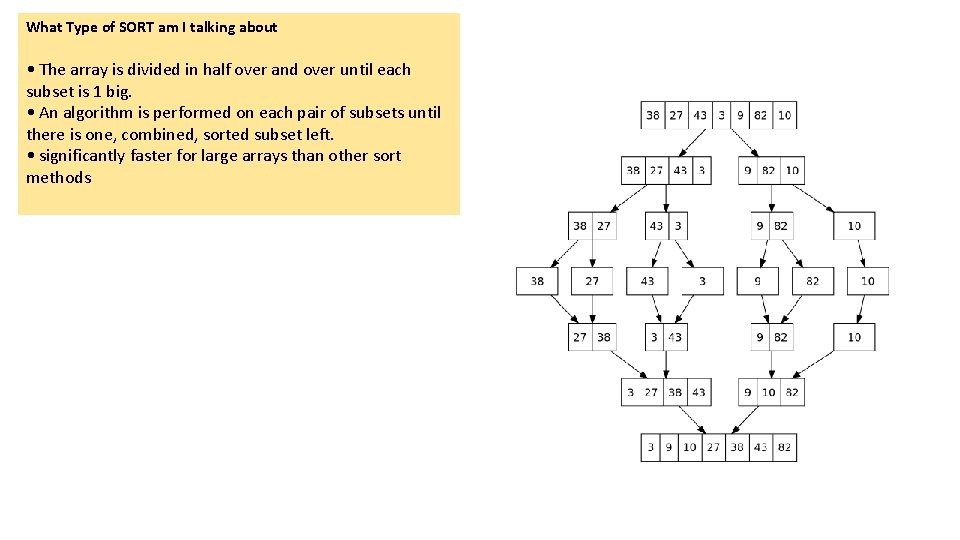What Type of SORT am I talking about • The array is divided in half over and over until each subset is 1 big. • An algorithm is performed on each pair of subsets until there is one, combined, sorted subset left. • significantly faster for large arrays than other sort methodsExplain the following Data Type Variable Integer Constant Assignment Description Real Character String Boolean WHAT IS CASTING: Programming construct Description Sequence WHAT IS an 1 D Array: Selection What is an 2 D Array: Iteration 1 2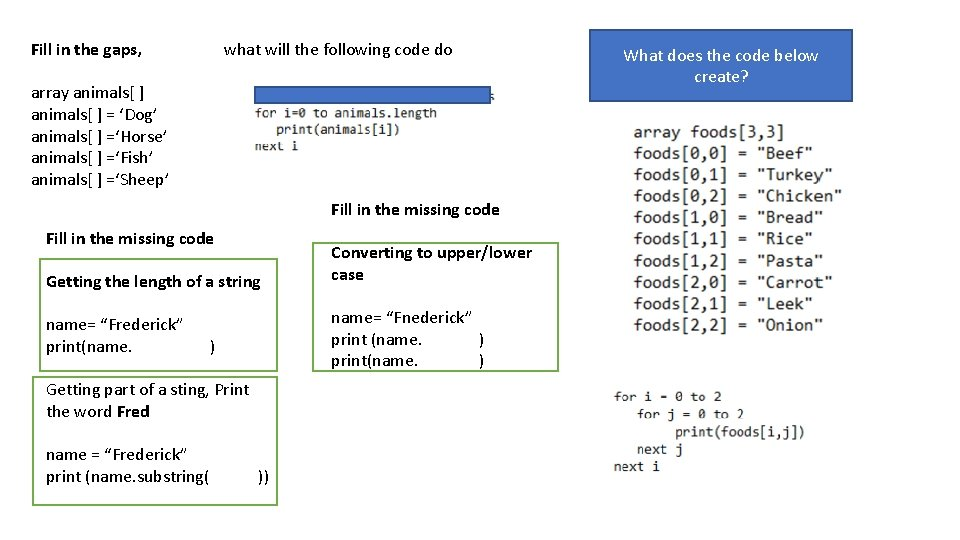Fill in the gaps, what will the following code do array animals[ ] = ‘Dog’ animals[ ] =‘Horse’ animals[ ] =‘Fish’ animals[ ] =‘Sheep’ Fill in the missing code Getting the length of a string name= “Frederick” print(name. name= “Fnederick” print (name. ) print(name. ) ) Getting part of a sting, Print the word Fred name = “Frederick” print (name. substring( Converting to upper/lower case )) What does the code below create?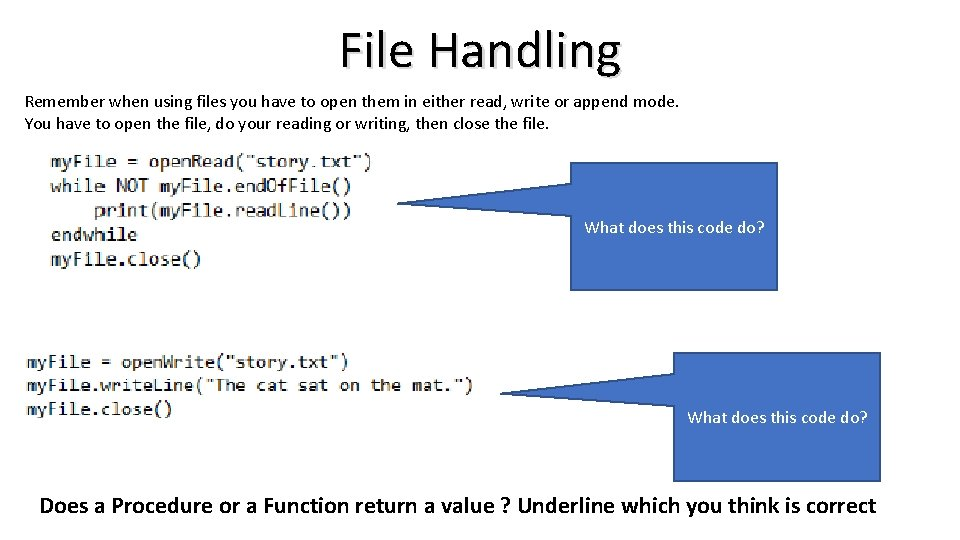File Handling Remember when using files you have to open them in either read, write or append mode. You have to open the file, do your reading or writing, then close the file. What does this code do? Does a Procedure or a Function return a value ? Underline which you think is correctProcedures and Functions procedure hello(name) print(“Hello “ + ) Endprocedure function doubler(number) return double * 2 End function fill in the missing code What is this part of the function called Name the operator Operator == * != / < - > MOD >= DIV Power of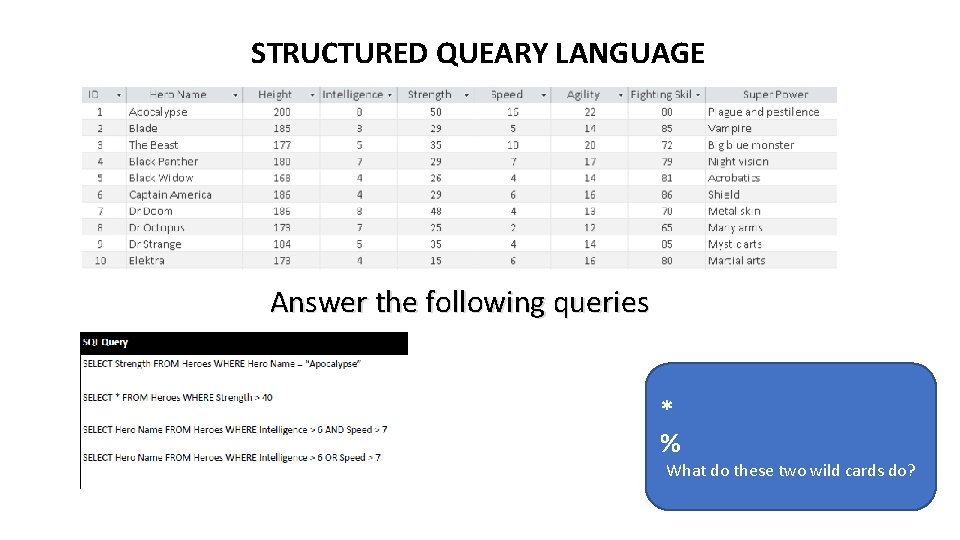STRUCTURED QUEARY LANGUAGE Answer the following queries * % What do these two wild cards do?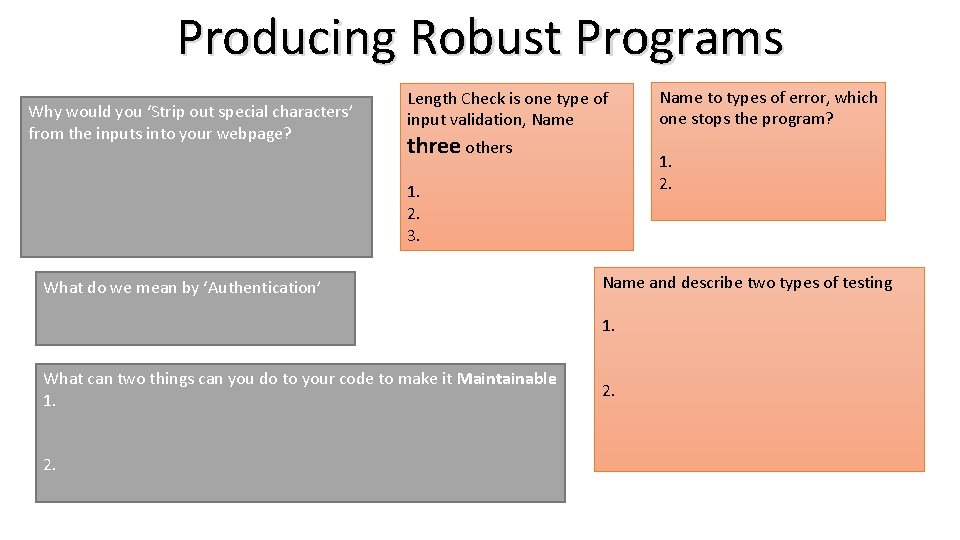Producing Robust Programs Why would you ‘Strip out special characters’ from the inputs into your webpage? Length Check is one type of input validation, Name three others 1. 2. 3. What do we mean by ‘Authentication’ Name and describe two types of testing 1. What can two things can you do to your code to make it Maintainable 1. 2. Name to types of error, which one stops the program? 2.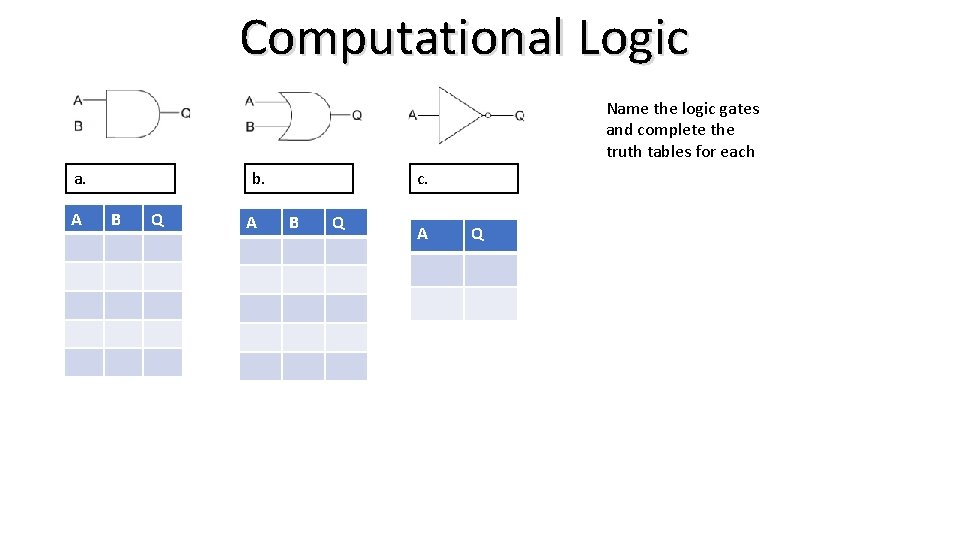Computational Logic Name the logic gates and complete the truth tables for each a. A b. B Q A c. B Q A Q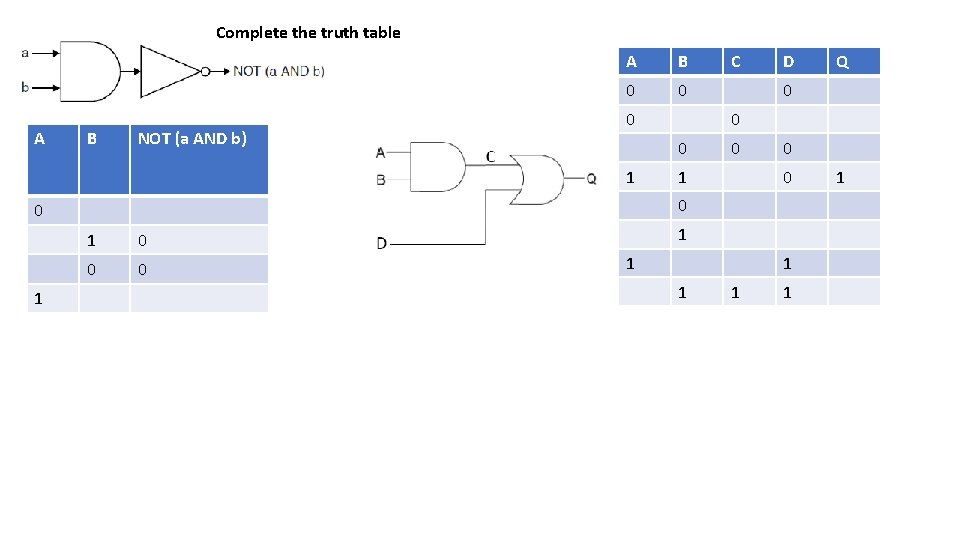Complete the truth table A B NOT (a AND b) A B 0 0 0 1 0 0 0 Q 0 0 1 D 0 0 1 C 1 1 1 1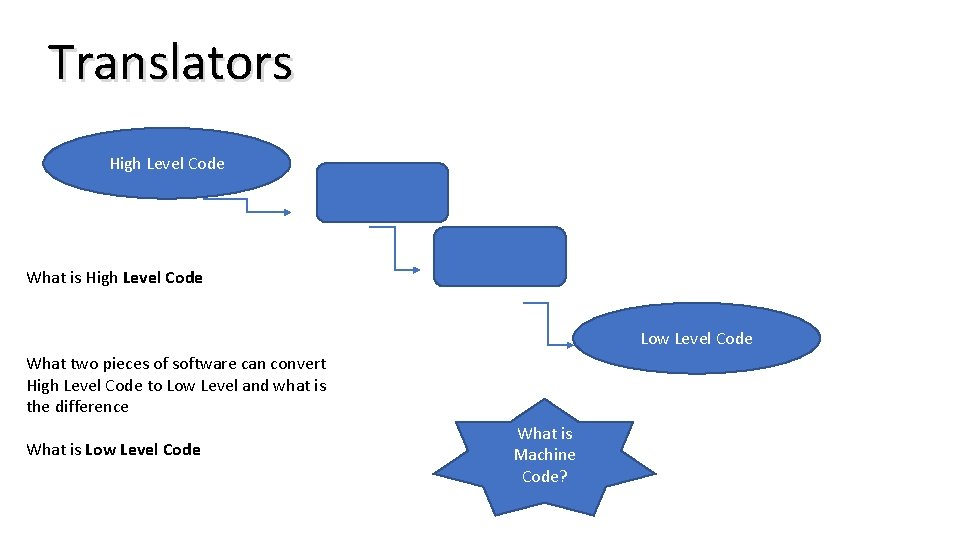Translators High Level Code What is High Level Code Low Level Code What two pieces of software can convert High Level Code to Low Level and what is the difference What is Low Level Code What is Machine Code?IDEs The Integrated Developer Environment is the program you use to write your code in. An IDE has lots of features that make it easier to develop software. Name and explain 3 features of an IDE 1, 2, 3,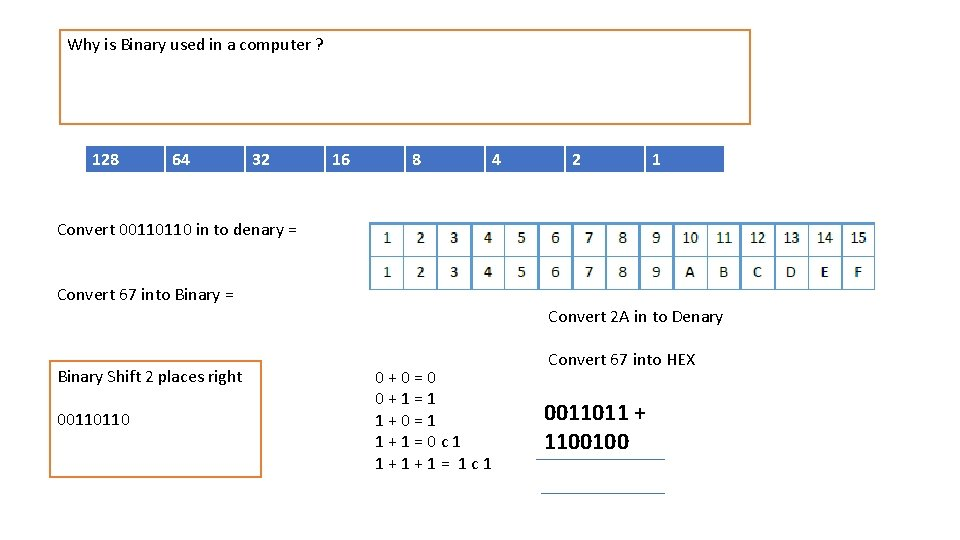Why is Binary used in a computer ? 128 64 32 16 8 4 2 1 Convert 00110110 in to denary = Convert 67 into Binary = Binary Shift 2 places right 00110110 Convert 2 A in to Denary 0+0=0 0+1=1 1+0=1 1+1=0 c 1 1+1+1= 1 c 1 Convert 67 into HEX 0011011 + 1100100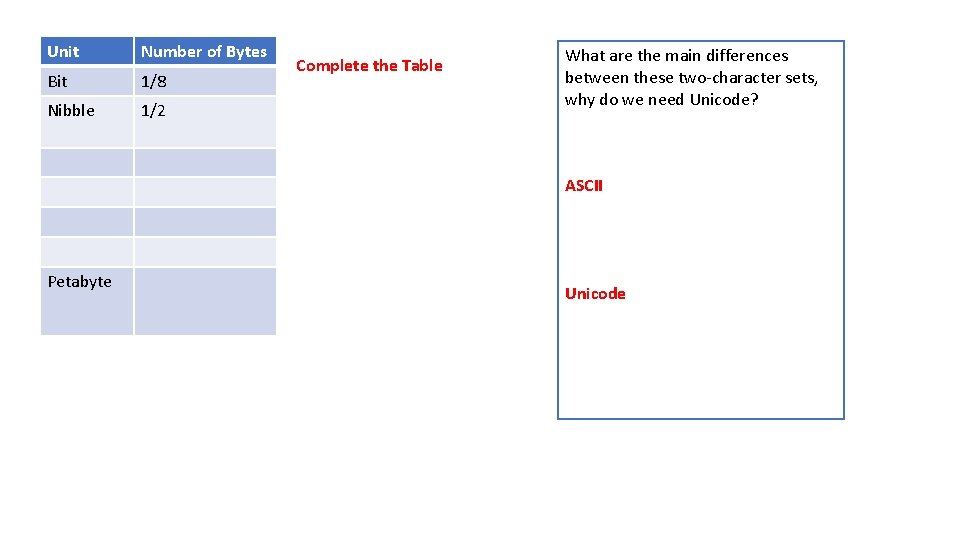Unit Number of Bytes Bit 1/8 Nibble 1/2 Complete the Table What are the main differences between these two-character sets, why do we need Unicode? ASCII Petabyte UnicodeSound • What do we do to turn Sound into Binary? Compression Lossy • Why would we get a large File Size? • What is the Amplitude of a sound wave? Images • What is the BIT RATE? • What is a Bitmap • What is the Resolution of an image? • What is the Colour Dept of an image? • Why would we get a large file size? • Name 3 things stored in an images meta data 1 2 3 Lossless